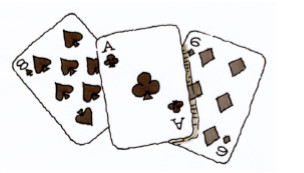### Home > MC2 > Chapter 1 > Lesson 1.2.1 > Problem1-47

1-47.

A standard deck of playing cards has four suits (symbols) in two colors: diamonds and hearts are red; clubs and spades are black. Each suit has $13$ cards: an ace, the numbers two through ten, a jack, a queen, and a king.1. What is $\text{P}$(black)?

P(black) means that you are finding the probability of drawing a black card from the deck.

How many black cards (spades and clubs) are there? How many cards are there total? Use a fraction in your answer.

Remember, the total number of cards is the denominator and the number of black cards is the numerator.

$\frac{26}{52}=\frac{1}{2}$

1. What is $\text{P}$(ace)?

How many aces are there in a deck of cards? How many cards are there total?

Remember, there are $4$ suits with $1$ ace in each suit.

1. What is $\text{P}$(club)?

How many clubs are there in a deck of cards? How many cards are there total?

Remember, each suit has $13$ cards.

1. If you drew a card from the deck and then replaced it, and repeated this $100$ times, about how many times would you expect to draw a face card (king, queen, or jack)?Hint (d):

What is $\text{P}$(face)?

What is $\text{P}$(face) one hundred times? $\text{P}$(face) x $100 =$ ?

About $22$ or $23$ times